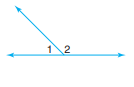# ?Use the terms adjacent angles, linear pair, or neither to describe angles $$1$$ and

Chapter 3, Problem 3

#### (choose chapter or problem)

Use the terms adjacent angles, linear pair, or neither to describe angles $$1$$ and $$2$$ in as many ways as possible.Equation Transcription:Text Transcription:

1

2

Unfortunately, we don't have that question answered yet. But you can get it answered in just 5 hours by Logging in or Becoming a subscriber.